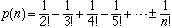My name: William J. Ricciardi
Level of question: college
Who is asking: a 7th grade math teacher

I gave a matching quiz the other day and one of my students got all 9 incorrect. That got me thinking......What is the probability that someone could get all 9 questions incorrect? I teach 7th grade math and it's been a long time since my Probability and Statistics course. If possible, please explain you answer so that it might jog my memory.

Hi William,

This is a really interesting problem. It is sometimes called the hatcheck problem. A hatcheck has n hats that get hopelessly scrambled. The hats are returned at random. What is the probability that every person gets the wrong hat? Let's, however, stick with your situation.
One reason this is an intriguing problem is that some people feel that for n large this probability is small while others feel it is large. With a little work you can show that for a matching test with n questions, if a student completes the test by guessing then the probability that he or she gets no answer correct iswhere n! = nx(n-1)x(n-2)x(n-3)x...x3x2x1. A table of values helps show what happens as n increases.

Number of
questions
n
Probability of
no matching correct
p(n)
20.50000
30.33333
40.37500
50.36667
60.36806
70.36786
80.36788
90.36788

As n gets large p(n) is neither very small nor very large. In fact, for n at least 6, p(n) is essentially constant. As the number of questions, n, increases p(n) approaches 1/e = 0.367879... The number e, the base for the natural logarithms, is approximately 2.71828183...
So, if your students were only guessing, you would expect approximately 36.9% of them to get all 9 questions incorrect.

Cheers,
Penny

Go to Math Central